Itâ€™s been a long time coming, but finally I get the time to explain the technique I came up with for drawing shader-based dynamic 2D shadows.

If you want to dive right into the code, you can go to the sampleâ€™s page.

Still here? Ok then, letâ€™s get started.

The core of the technique is drawing all the possible shadow casters around a light into a texture, and then using a few shaders to turn this image into one containing all shadows cast in the scene. First, for a general view, consider the following scene.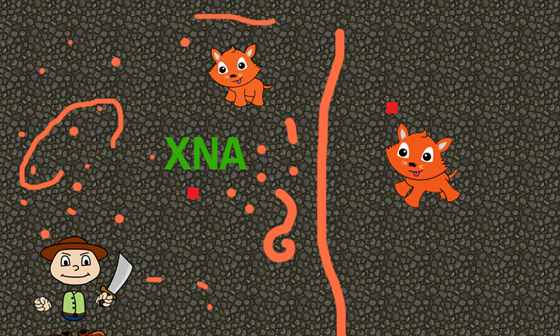Out goal is to have all objects cast shadows, with the two orange squares being the sources of light.

The first step is to build for each light a image of all the casters around it. This basically means drawing all objects with a black color into a texture, centered around the light. The technical side of this should be pretty trivial, and can also be seen in the code.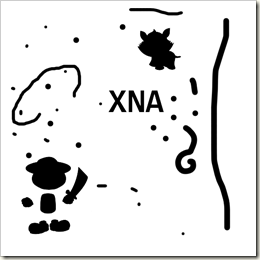The tricky part comes next and this is the main part of the technique. I will explain the whole process a little later, but for now just know that the technique I use takes as input these â€œcaster mapsâ€ and produces a â€œshadow imageâ€, as seen below.

Having these two shadow images, it is now easy to blend them together, using the desired light color for each, and then blending the result over the â€œgroundâ€.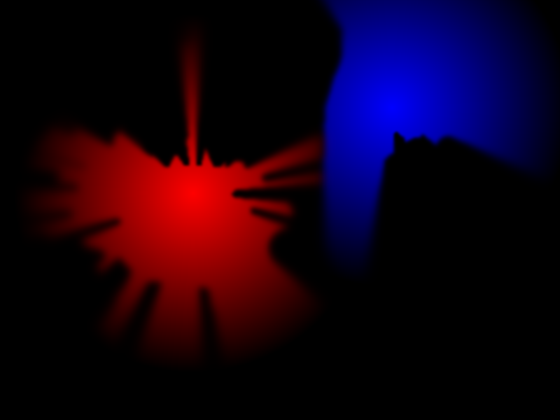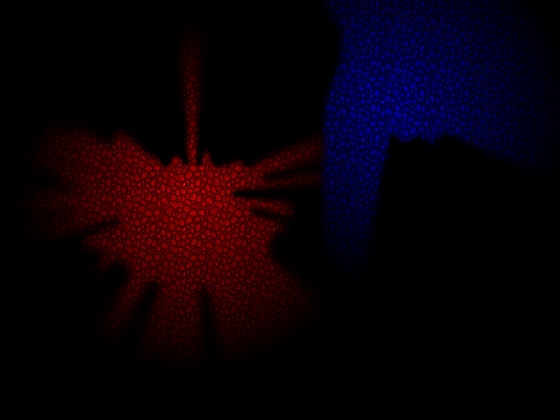In the end, drawing the items in the scene leads to our desired result.Most of these steps should be fairly easy. The problem comes in getting from the image containing all the shadow casters to the image containing the shadows themselves.

The technique is really not that complicated, so Iâ€™ll explain each step, together with shader code and images. So letâ€™s start with the beginning, namely the shadow casters image.1. Starting from this, for each non-transparent pixel (non-white in the illustration), I output the distance from the center of the texture to that pixel (i.e. the distance from the light source to the pixel).

```float4 ComputeDistancesPS(float2 TexCoord : TEXCOORD0) : COLOR0
{
float4 color = tex2D(inputSampler, TexCoord);
Â  Â  //compute distance from center
float distance = color.a&gt;0.3f?length(TexCoord - 0.5f):1.0f;
//save it to the Red channel
distance *= renderTargetSize.x;
return float4(distance,0,0,1);
}
```

One thing to notice is that I multiply by the size of the render target and save to a floating point format. I do this in order to preserve precision. You could store this as a value between 0 and 1, and write it into a render target with the Color surface format, but this can lead to some imprecision when using large lights.

The results looks something like below (shown in grayscale for easy visualization).2. In the next step, I divide the image into four quadrants around the light (center of this image), and distort it in such a way that all â€œlight raysâ€ (which are at the moment spreading in all directions from the light) are positioned in parallel. This is much easier to understand with an image, as seen below.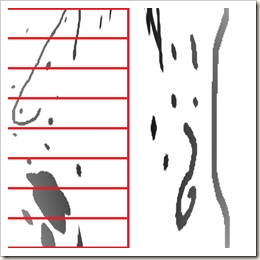In the pictures above, the quadrant to the left (and the one to the right) has been distorted so it takes up half of the image, and all pixels are placed as if the rays starting from the light are parallel. The reason I am doing this will be obvious in the next step. Since I need this data for all the quadrants, I make the same operation for the vertical direction (rotating the result so itâ€™s also horizontal).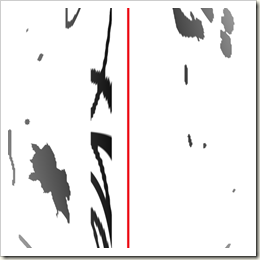I know the text doesnâ€™t make as much sense as I would like but hopefully the images illustrate what I mean. Finally, to reduce memory usage and to perform more computations in parallel, I store the result of the horizontal distortion in the red channel, and the result of the vertical distortion in the green channel.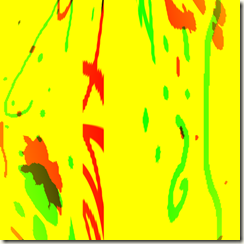I know it doesnâ€™t look like much, but know that all the data we need for all directions is now stored in a single texture. The code that achieves this step is the shader function below. This code can probably be simplified (to avoid the conversions between the [0,1] and [-1,1] domains, but I find it clearer this way.

```float4 DistortPS(float2 TexCoord : TEXCOORD0) : COLOR0
{
//translate u and v into [-1 , 1] domain
float u0 = TexCoord.x * 2 - 1;
float v0 = TexCoord.y * 2 - 1;

//then, as u0 approaches 0 (the center), v should also approach 0
v0 = v0 * abs(u0);
//convert back from [-1,1] domain to [0,1] domain
v0 = (v0 + 1) / 2;
//we now have the coordinates for reading from the initial image
float2 newCoords = float2(TexCoord.x, v0);

//read for both horizontal and vertical direction and store them in separate channels

float horizontal = tex2D(inputSampler, newCoords).r;
float vertical = tex2D(inputSampler, newCoords.yx).r;
return float4(horizontal,vertical ,0,1);
}```

3. Now that we have the distorted images of the distances from the light along each ray going out from the light, itâ€™s time to compute the minimum along each of these rays. I do this by successive reduction of the image along the horizontal direction, until a texture of width 2 is obtained. This texture is something very similar to a shadow map in 3D, in that it contains for each ray, the minimum distance from the light where a shadow caster is present.This step is also currently the bottleneck of the algorithm, since reducing a texture of 512*512 to a size of 2*512 requires about 9 passes (the dimension is reduced by a factor of 2 through each pass). The reduction shader is a simple one:

```float4 HorizontalReductionPS(float2 TexCoord : TEXCOORD0) : COLOR0
{
float2 color = tex2D(inputSampler, TexCoord);
float2 colorR = tex2D(inputSampler, TexCoord + float2(TextureDimensions.x,0));
float2 result = min(color,colorR);
return float4(result,0,1);
}```

At this point, it should be clear why I distorted the pixels in such a way (in order to be able to apply a horizontal reduction) and why the vertical components were also rotated and stored in a separate channel. Basically, we make the reductions on all directions at the same time.

Note: because this is the bottleneck, an option is to store the caster texture and do all operation on half the size of the actual light area. Thus for a light area of 512*512 you could make the processing on textures of 256*256. This way you loose some crispiness (not always a bad thing when it comes to shadows) but you also gain some performance.

4. At this moment, we can generate the shadow image. We draw the area surrounding the light, and for each pixel, we compare the distance between this pixel and the light to the distance stored in the shadow map along the same ray. This information tells us if we are in front or behind the shadow caster.

```float4 DrawShadowsPS(float2 TexCoord: TEXCOORD0) : COLOR0
{
// distance of this pixel from the center
float distance = length(TexCoord - 0.5f);
distance *= renderTargetSize.x;

//apply a 2-pixel bias
distance -=2;

//distance stored in the shadow map
//coords in [-1,1]
float nY = 2.0f*( TexCoord.y - 0.5f);
float nX = 2.0f*( TexCoord.x - 0.5f);

//we use these to determine which quadrant we are in
if(abs(nY)&lt;abs(nX))
{
}
else
{
}

//if distance to this pixel is lower than distance from shadowMap,
//then we are not in shadow
float light = distance < shadowMapDistance ? 1:0;
float4 result = light;
result.b = length(TexCoord - 0.5f);
result.a = 1;
return result;
}```

The functions that read from the shadow map make an inverse transformation of coordinates in order to read from the proper location in the shadowmap.

```float GetShadowDistanceH(float2 TexCoord)
{
float u = TexCoord.x;
float v = TexCoord.y;

u = abs(u-0.5f) * 2;
v = v * 2 - 1;
float v0 = v/u;
v0 = (v0 + 1) / 2;

float2 newCoords = float2(TexCoord.x,v0);
//horizontal info was stored in the Red component
}

{
float u = TexCoord.y;
float v = TexCoord.x;

u = abs(u-0.5f) * 2;
v = v * 2 - 1;
float v0 = v/u;
v0 = (v0 + 1) / 2;

float2 newCoords = float2(TexCoord.y,v0);
//vertical info was stored in the Green component
}
```

(Note: in the source code, you might notice a commented out version of the DrawShadowsPS function. That one was using three taps in order to smooth out the aliasing of the shadows, quite similar to what PCF method for 3D shadow maps does. This was left out because the smoothing through blur is much better looking)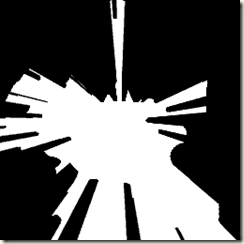Another thing to notice is that we store the distance from the center in the Blue component of the result. This is useful when we want to smooth out the shadows by applying Gaussian blur, with radius depending on the distance from the light. Thus we obtain harder shadows closer to the light source, and softer shadows further away.

5. Next in my implementation, I apply a horizontal blur, followed by a vertical blur. The code for this is not too special, except for the fact that the blur radius depends on the distance from the light. All shader source can be seen in the attached archive, and I will not reproduce the blur shader here. When doing the final blur step, I also add some attenuation to the light, and the result is finally the one we were looking for.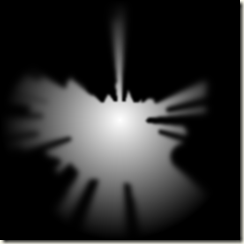And this concludes the explanations about the algorithm I used for the shader-based soft 2D shadows. I hope my explanations were clear enough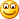You can download the source code from the sampleâ€™s page, found here.

Until next time, have fun coding!

• http://ciio.unab.edu.co/gti/seminario/user/view.php?id=13487&course=1 http://150.254.69.198/moodle/user/view.php?id=7691&course=1

If a do, accompanied by a person will already have to acknowledge because Life insurance quotes is able to aspect accessible basically
infinite punishment.

• http://avoidcowboybuilders.wordpress.com Avoidcowboybuilders.Wordpress.Com